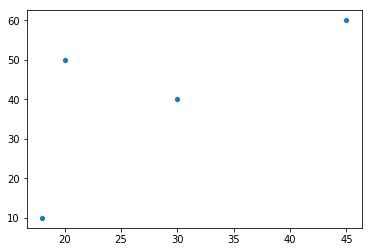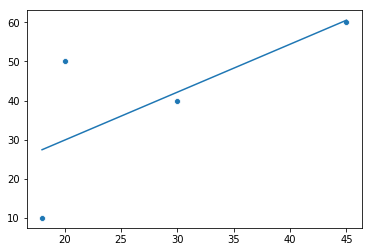In :
#import libraries
import sklearn
import numpy as np
import seaborn as sns
from sklearn.linear_model import LinearRegression


# Create our x and y value arrays and plot them¶

In :
#x = ages
#y = spend per week
x = np.array([18, 20, 30, 45])
y = np.array([10, 50, 40, 60])

#plot our two generated arrays on scatterplot
sns.scatterplot(x,y)

Out:
<matplotlib.axes._subplots.AxesSubplot at 0x1a15de9eb8># reshape the data & fit it to the model.¶

In :
model = LinearRegression()

#reshaped to x rows and 1 columns. the -1 fills in the blank (num of rows)
x = x.reshape(-1, 1)
x.shape

#fit is the 'learning bit' - it's fitting the line to the data (fitting the formula to the data)
#model.coef_, model.intercept_ are the a & b from y = ax + b
model.fit(x,y)

#these are the parameters from y = ax + b (a = gradient, b = intercept)
print(model.coef_)
print(model.intercept_)

[1.22605364]
5.363984674329487


# Feed x into the model to predict y¶

In :
#predict y value based on x
y_pred = model.predict(x)

#print the predictions for the x's
print(y_pred)

[27.43295019 29.88505747 42.14559387 60.53639847]


# Plot the original x & y and the new x & y predicted (prediction as line)¶

In :
#print the estimates and the actuals on the same chart
#ravel means remove shape - just give me the datapoints
sns.scatterplot(x.ravel(),y)
sns.lineplot(x.ravel(), y_pred)

Out:
<matplotlib.axes._subplots.AxesSubplot at 0x1a15eff5c0># Print the model accuracy score¶

In :
#model score (1 = 100% correct. 0 = 0% correct)
model.score(x,y)

Out:
0.4904214559386973

# Example 2¶

In :
from sklearn.datasets import load_breast_cancer
data = load_breast_cancer()

x = data.data
y = data.target
z = data.target_names

model = LinearRegression().fit(x,y)
model.score(x,y)

Out:
0.7743246526421793
In :
import pandas as pd
df = pd.DataFrame(x, columns=data.feature_names)
df.head()

Out:
mean radius mean texture mean perimeter mean area mean smoothness mean compactness mean concavity mean concave points mean symmetry mean fractal dimension ... worst radius worst texture worst perimeter worst area worst smoothness worst compactness worst concavity worst concave points worst symmetry worst fractal dimension
0 17.99 10.38 122.80 1001.0 0.11840 0.27760 0.3001 0.14710 0.2419 0.07871 ... 25.38 17.33 184.60 2019.0 0.1622 0.6656 0.7119 0.2654 0.4601 0.11890
1 20.57 17.77 132.90 1326.0 0.08474 0.07864 0.0869 0.07017 0.1812 0.05667 ... 24.99 23.41 158.80 1956.0 0.1238 0.1866 0.2416 0.1860 0.2750 0.08902
2 19.69 21.25 130.00 1203.0 0.10960 0.15990 0.1974 0.12790 0.2069 0.05999 ... 23.57 25.53 152.50 1709.0 0.1444 0.4245 0.4504 0.2430 0.3613 0.08758
3 11.42 20.38 77.58 386.1 0.14250 0.28390 0.2414 0.10520 0.2597 0.09744 ... 14.91 26.50 98.87 567.7 0.2098 0.8663 0.6869 0.2575 0.6638 0.17300
4 20.29 14.34 135.10 1297.0 0.10030 0.13280 0.1980 0.10430 0.1809 0.05883 ... 22.54 16.67 152.20 1575.0 0.1374 0.2050 0.4000 0.1625 0.2364 0.07678

5 rows × 30 columns